Home » 4 Simple Ways to Split a Decision Tree in Machine Learning

# 4 Simple Ways to Split a Decision Tree in Machine Learning

## Overview

• How do you split a decision tree? What are the different splitting criteria when working with decision trees?
• Learn all about decision tree splitting methods here and master a popular machine learning algorithm

## Introduction

Decision trees are simple to implement and equally easy to interpret. I often lean on decision trees as my go-to machine learning algorithm, whether I’m starting a new project or competing in a hackathon.

And decision trees are idea for machine learning newcomers as well! But the questions you should ask (and should know the answer to) are:

• How do you split a decision tree?
• What are the different splitting criteria?
• What is the difference between Gini and Information Gain?

If you are unsure about even one of these questions, you’ve come to the right place! Decision Tree is a powerful machine learning algorithm that also serves as the building block for other widely used and complicated machine learning algorithms like Random Forest, XGBoost, and LightGBM. You can imagine why it’s important to learn about this topic!Modern-day programming libraries have made using any machine learning algorithm easy, but this comes at the cost of hidden implementation, which is a must-know for fully understanding an algorithm. Another reason for this infinite struggle is the availability of multiple ways to split decision tree nodes adding to further confusion.

Have you ever encountered this struggle? Failed to find a solution? In this article, I will explain 4 simple methods for splitting a node in a decision tree.

I assume familiarity with the basic concepts in regression and decision trees. Here are two free and popular courses to quickly learn or brush up on the key concepts:

## Basic Decision Tree Terminologies

Let’s quickly revise the key terminologies related to decision trees which I’ll be using throughout the article.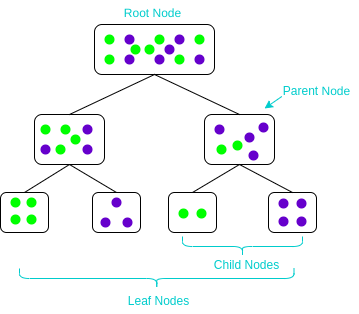• Parent and Child Node: A node that gets divided into sub-nodes is known as Parent Node, and these sub-nodes are known as Child Nodes. Since a node can be divided into multiple sub-nodes, therefore a node can act as a parent node of numerous child nodes
• Root Node: The top-most node of a decision tree. It does not have any parent node. It represents the entire population or sample
• Leaf / Terminal Nodes: Nodes that do not have any child node are known as Terminal/Leaf Nodes

## What is Node Splitting in a Decision Tree & Why is it Done?

Before learning any topic, I believe it is essential to understand why you’re learning it. That helps in understanding the goal of learning a concept. So let’s understand why to learn about node splitting in decision trees.

Since you all know how extensively decision trees are used, there is no denying the fact that learning about decision trees is a must. A decision tree makes decisions by splitting nodes into sub-nodes. This process is performed multiple times during the training process until only homogenous nodes are left. And it is the only reason why a decision tree can perform so well. Therefore, node splitting is a key concept that everyone should know.

Node splitting, or simply splitting, is the process of dividing a node into multiple sub-nodes to create relatively pure nodes. There are multiple ways of doing this, which can be broadly divided into two categories based on the type of target variable:

1. Continuous Target Variable
• Reduction in Variance
2. Categorical Target Variable
• Gini Impurity
• Information Gain
• Chi-Square

In the upcoming sections, we’ll look at each splitting method in detail. Let’s start with the first method of splitting – reduction in variance.

## Decision Tree Splitting Method #1: Reduction in Variance

Reduction in Variance is a method for splitting the node used when the target variable is continuous, i.e., regression problems. It is so-called because it uses variance as a measure for deciding the feature on which node is split into child nodes.Variance is used for calculating the homogeneity of a node. If a node is entirely homogeneous, then the variance is zero.

Here are the steps to split a decision tree using reduction in variance:

1. For each split, individually calculate the variance of each child node
2. Calculate the variance of each split as the weighted average variance of child nodes
3. Select the split with the lowest variance
4. Perform steps 1-3 until completely homogeneous nodes are achieved

The below video excellently explains the reduction in variance using an example:

## Decision Tree Splitting Method #2: Information Gain

Now, what if we have a categorical target variable? Reduction in variation won’t quite cut it.

Well, the answer to that is Information Gain. Information Gain is used for splitting the nodes when the target variable is categorical. It works on the concept of the entropy and is given by: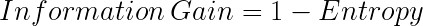Entropy is used for calculating the purity of a node. Lower the value of entropy, higher is the purity of the node. The entropy of a homogeneous node is zero. Since we subtract entropy from 1, the Information Gain is higher for the purer nodes with a maximum value of 1. Now, let’s take a look at the formula for calculating the entropy:Steps to split a decision tree using Information Gain:

1. For each split, individually calculate the entropy of each child node
2. Calculate the entropy of each split as the weighted average entropy of child nodes
3. Select the split with the lowest entropy or highest information gain
4. Until you achieve homogeneous nodes, repeat steps 1-3

Here’s a video on how to use information gain for splitting a decision tree:

## Decision Tree Splitting Method #3: Gini Impurity

Gini Impurity is a method for splitting the nodes when the target variable is categorical. It is the most popular and the easiest way to split a decision tree. The Gini Impurity value is: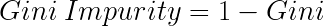Wait – what is Gini?

Gini is the probability of correctly labeling a randomly chosen element if it was randomly labeled according to the distribution of labels in the node. The formula for Gini is: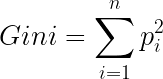And Gini Impurity is: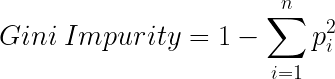Lower the Gini Impurity, higher is the homogeneity of the node. The Gini Impurity of a pure node is zero. Now, you might be thinking we already know about Information Gain then, why do we need Gini Impurity?

Gini Impurity is preferred to Information Gain because it does not contain logarithms which are computationally intensive.

Here are the steps to split a decision tree using Gini Impurity:

1. Similar to what we did in information gain. For each split, individually calculate the Gini Impurity of each child node
2. Calculate the Gini Impurity of each split as the weighted average Gini Impurity of child nodes
3. Select the split with the lowest value of Gini Impurity
4. Until you achieve homogeneous nodes, repeat steps 1-3

And here’s Gini Impurity in video form:

## Decision Tree Splitting Method #4: Chi-Square

Chi-square is another method of splitting nodes in a decision tree for datasets having categorical target values. It can make two or more than two splits. It works on the statistical significance of differences between the parent node and child nodes.

Chi-Square value is: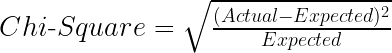Here, the Expected is the expected value for a class in a child node based on the distribution of classes in the parent node, and Actual is the actual value for a class in a child node.

The above formula gives us the value of Chi-Square for a class. Take the sum of Chi-Square values for all the classes in a node to calculate the Chi-Square for that node. Higher the value, higher will be the differences between parent and child nodes, i.e., higher will be the homogeneity.

Here are the steps to split a decision tree using Chi-Square:

1. For each split, individually calculate the Chi-Square value of each child node by taking the sum of Chi-Square values for each class in a node
2. Calculate the Chi-Square value of each split as the sum of Chi-Square values for all the child nodes
3. Select the split with higher Chi-Square value
4. Until you achieve homogeneous nodes, repeat steps 1-3

Of course, there’s a video explaining Chi-Square in the context of a decision tree:

## End Notes

Now, you know about different methods of splitting a decision tree. In the next steps, you can watch our complete playlist on decision trees on youtube. Or, you can take our free course on decision trees here.

I have also put together a list of fantastic articles on decision trees below:

You can also read this article on our Mobile APP•MANEESH KUMAR SINGH says:
•Abhishek Sharma says: